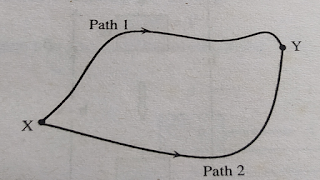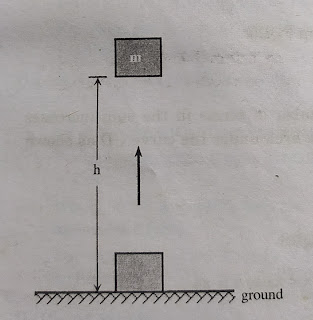### Mechanics//Work Energy and Power//Conservative and Non Conservative Forces//Examples of Conservative and Non Conservative Forces//Properties of Conservative Forces//Potential Energy//Gravitational Potential Energy

1. Conservative and Non-conservative               Forces

A force is called as conservative if the work done by or against the force in moving a body depends only on the initial and final positions of the body, and not on the nature of path followed between the initial and the final positions i.e. amount of work done is independent of its path followed. It suggests that work done by or against a conservative force in moving a body over any path between fixed initial and final positions will be the same.Figure : Forceful man

Magnetic force, electrostatic force, gravitational force, force in an elastic spring, etc. are examples of conservative force. Likewise, all the central forces are conservative forces.Figure: Work done by conservative force between X and Y.

Consider for example: In case of gravitational force, if we take work done in moving the body from x to y, against gravity as negative, then work done in moving the body from Y to X, by gravity has to be taken as positive. they are equal and opposite i.e.

W(XY) = - W(YX)

or,    W(XY)+ W(YX)=0

This indicates that, the total amount of work done in a closed path in conservative force field (gravitational field) is zero.

2. Properties of Conservative Forces

(i) Work done by or against a conservative force, in moving a body from one position to the other depends only on the initial and final positions of the body.

(ii) Work done by or against a conservative force does not rely on the nature of the path followed by the body in going from initial position to the final position.

(iii) Work done by or against a conservative force in moving a body in a closed path (where the final and initial position coincides) is always zero.

3. Non-Conservative Forces
A force is called as non-conservative, if work done by or against the force in moving a body from one position to another, depends on the path followed between these two positions.

Consider for example: All the frictional forces are non-conservative forces. If a body is displaced from position A to position B on a rough table, work done against frictional force will depend on the length of the path between A and B and not only on the positions A and B.
Also, if the body is brought back to its initial position work has to be done against the frictional forces, which oppose the motion always. Hence net work done against the frictional forces in moving a body over a closed path is not zero.

For example: Induction force in a cyclotron is a non-conservative force.

4. Potential Energy

It may be defined as the energy possessed by a body due to its position or due to its configuration. For examples, a compressed spring, an object above the ground, a compressed fluid, a stretched rubber band etc.

5. Gravitational Potential Energy
For example a body of mass m on the surface of the earth. The earth attracts it with a force equal to its weight mg.
If it is taken to a height h above the ground as shown in figure below.Figure: Potential energy of a body at height, h is mgh.

Work done against the gravity is

W = mgh

This work done will be stored in the body as gravitational potential energy

So, gravitational potential energy, mgh.